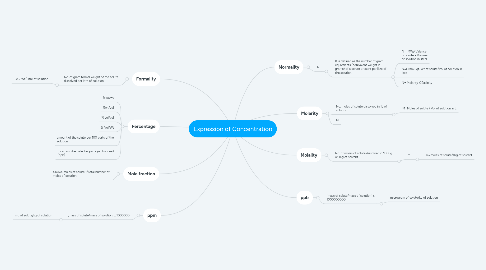# Expression of ConcentrationRMK1

Get Started. It's FreeExpression of Concentration## 1. Normality

### 1.1. N

1.1.1. It is defined as the number of gram equivalents (equivalent weight in grams) of a solute present per litre of the solution.

1.1.1.1. N = MW/(Valence of solute x Volume of solution in liter)

1.1.1.2. N=Gram Eqt. Wt of solute/Vol of solution in litre

1.1.1.3. N= Molarity X Basicity

## 2. Molarity

### 2.1. No. moles of solute dissolved in 1L of solution

2.1.1. M=Moles of solute / Vol of solution in L

## 3. Formality

### 3.1. No. of gram formal weight of the solute dissolved per litre of solution

3.1.1. F=GFWt/Litre of solution

## 6. Molality

### 6.1. No. of moles of solute dissolved in 1000g or 1Kg of solvent

6.1.1. m

6.1.1.1. m=moles of solute/1Kg of solvent

## 7. ppm

### 7.1. [mass of solute/mass of solution] x 1000000

7.1.1. mg of solute/kg of solution

## 8. ppb

### 8.1. [mass of solute/mass of solution] x 1000000000

8.1.1. microgram of solute/kg of solution